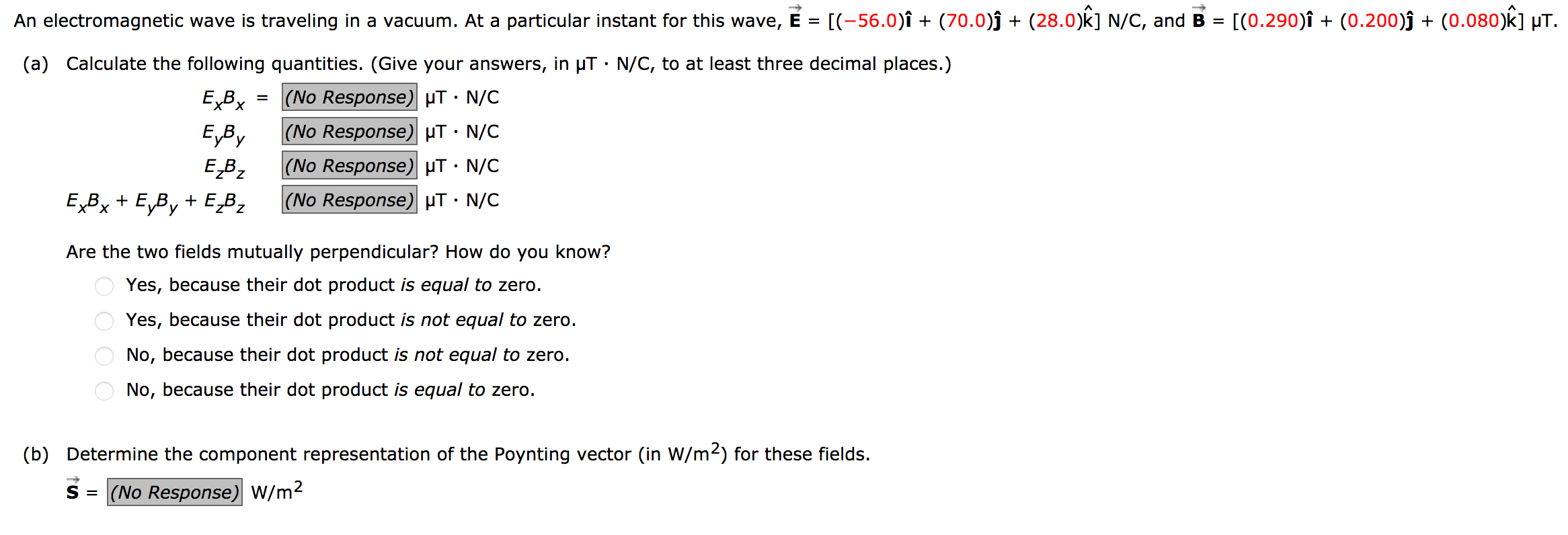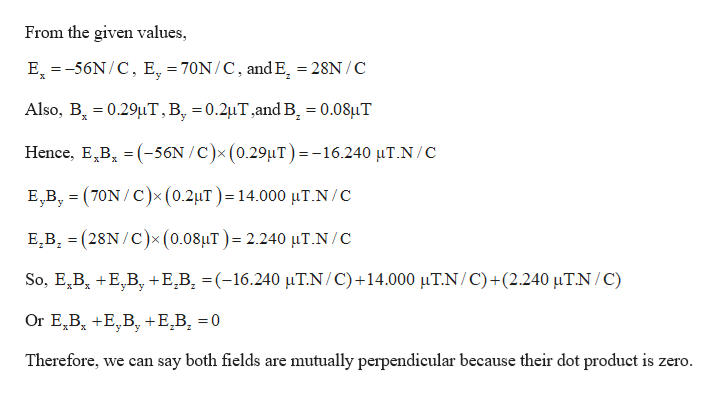# [(0.290)î + (0.200)ĵ + (0.080)k] µT.An electromagnetic wave is traveling in a vacuum. At a particular instant for this wave, E = [(-56.0)î + (70.0)ĵ + (28.0)k] N/C, and B =(a) Calculate the following quantities. (Give your answers, in µT · N/C, to at least three decimal places.)= (No Response) µT • N/C|(No Response) µT· N/C|(No Response) µT · N/CE,ByEBz|(No Response) µT · N/CEBx + E,By + E,B,Are the two fields mutually perpendicular? How do you know?Yes, because their dot product is equal to zero.Yes, because their dot product is not equal to zero.No, because their dot product is not equal to zero.No, because their dot product is equal to zero.(b) Determine the component representation of the Poynting vector (in W/m2) for these fields.S = (No Response) W/m2

Question
1 viewshelp_outlineImage Transcriptionclose[(0.290)î + (0.200)ĵ + (0.080)k] µT. An electromagnetic wave is traveling in a vacuum. At a particular instant for this wave, E = [(-56.0)î + (70.0)ĵ + (28.0)k] N/C, and B = (a) Calculate the following quantities. (Give your answers, in µT · N/C, to at least three decimal places.) = (No Response) µT • N/C |(No Response) µT· N/C |(No Response) µT · N/C E,By EBz |(No Response) µT · N/C EBx + E,By + E,B, Are the two fields mutually perpendicular? How do you know? Yes, because their dot product is equal to zero. Yes, because their dot product is not equal to zero. No, because their dot product is not equal to zero. No, because their dot product is equal to zero. (b) Determine the component representation of the Poynting vector (in W/m2) for these fields. S = (No Response) W/m2 fullscreen
check_circle

Step 1

Given:

Electric field vector, E = (-56)I + (70)j + (28)k N/C

Magnetic field vector, B = (0.29)I + (0.2)j + (0.08)k μT

Step 2

Calculating the give...help_outlineImage TranscriptioncloseFrom the given values, E, =-56N/C, E, = 70N/C, and E, = 28N /C 'y Also, B, = 0.29µT,B, =0.2µT,and B, = 0.08uT %3! %3D х Hence, E,B, = (-56N /C)x (0.29µT)=-16.240 µT.N/C E,B, = (70N / C)x(0.2µT)=14.000 µT.N/C E,B, = (28N /C)x(0.08µT)= 2.240 µT.N/C So, E,B, +E,B, +E,B¸ =(-16.240 µT.N/C)+14.000 µT.N/C)+(2.240 µT.N/C) Or E,B, +E,B, +E̟B, = 0 Therefore, we can say both fields are mutually perpendicular because their dot product is zero. fullscreen

### Want to see the full answer?

See Solution

#### Want to see this answer and more?

Solutions are written by subject experts who are available 24/7. Questions are typically answered within 1 hour.*

See Solution
*Response times may vary by subject and question.
Tagged in

### Science